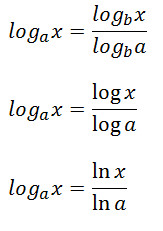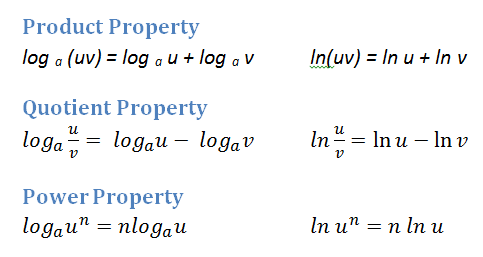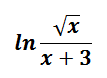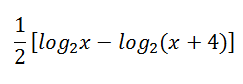# Properties of Logarithms

//Properties of Logarithms
Properties of Logarithms 2017-11-13T22:01:53+00:00

As you have probably noticed, your calculator only has two log buttons: the common log, log 10 and the natural log, log e or ln. Fortunately, by using the change of base theorem, you can evaluate a log of any base.

### Change of Base Theorem### Evaluate

Round the result to three decimal places.

log 6 9

Take the log of 9 divided by the log of 6. The result is 1.226.

log 2 14

Divide the log of 14 by the log of 2 The answer is 3.807.

log 1/4 12

You can also use the natural log instead of the common log. Take ln 12 / ln .25. The result is -1.792.

### Evaluate

Do not use a calculator.## Properties of LogarithmsBecause the answer to a logarithmic equation is the exponent in an exponential equation, it makes sense that logarithms should behave as exponents do.

### Expand

log 5 3x2y### Condense

3 ln(x – 5) + ln xPrecalculus with Limits – Larson & Hostetler p.239-245